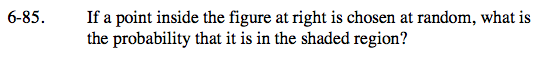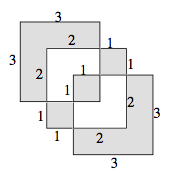### Home > A2C > Chapter 6 > Lesson 6.2.3 > Problem6-85

6-85.It might be easier if the individual 1 unit squares are separated.

Count up the total number of 1 unit squares.

Now count the number of shaded squares.Now use those two values to form the probability.

$\frac{13}{19}$